# Verbal Reasoning - Character Puzzles

### Exercise :: Character Puzzles - Character Puzzles 1

31.

Which one will replace the question mark ?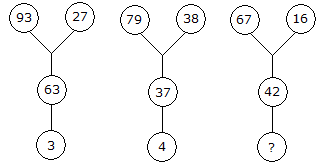A. 5 B. 6 C. 8 D. 9

Explanation:

93 - (27 + 3) = 63

79 - (38 + 4) = 37

Therefore, 67 - (16 + X) = 42

X = 9

32.

Which one will replace the question mark ?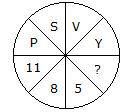A. 3 B. 2 C. 7 D. 6

Explanation:

Putting the position of the letters in reverse order

P = 11, S = 8, V = 5 and Y = 2.

33.

Which one will replace the question mark ?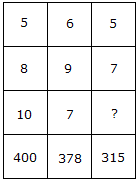A. 9 B. 5 C. 7 D. 3

Explanation:

5 x 8 x 10 = 400

and 6 x 9 x 7 = 378

Therefore 5 x 7 x ? = 315

? = 9.

34.

Which one will replace the question mark ?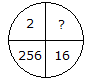A. 8 B. 32 C. 4 D. 16

Explanation:

(16)2 = 256

Therefore, (2)2 = 4.

35.

Which one will replace the question mark ?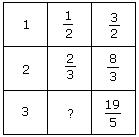A. 1/2 B. 2/3 C. 3/4 D. 4/5

Explanation:

From I row, 1 + (1/2) = 3/2

From II row, 2 + (2/3) = 8/3

From III row, 3 + ? = 19/5

? = (19/5) - 3

? = (4/5).